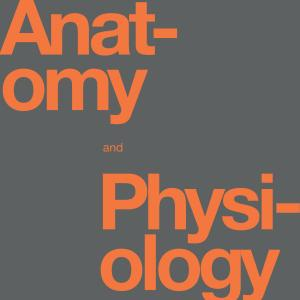# Fluid, Electrolyte and Acid-Base Balance

Source: OpenStax

Student Price: FREE

From Openstax: Anatomy and Physiology Chapter 26, this question pack explores fluid nutrition and the body's handling of acids and bases.

Fluid, Electrolyte and Acid-Base Balance 5

Solute contributes to the movement of water between cells and the surrounding medium by $\text{________}$.

A

osmotic pressure

B

hydrostatic pressure

C

Brownian movement

D

random motion

Fluid, Electrolyte and Acid-Base Balance 26.6

A cation has a(n) $\text{________}$ charge.

A

neutral

B

positive

C

alternating

D

negative

Fluid, Electrolyte and Acid-Base Balance 26.7

Interstitial fluid (IF) is $\text{________}$.

A

the fluid in the cytosol of the cells

B

the fluid component of blood

C

the fluid that bathes all of the body’s cells except for blood cells

D

the intracellular fluids found between membranes

Fluid, Electrolyte and Acid-Base Balance 26.8

The largest amount of water comes into the body via $\text{________}$.

A

metabolism

B

foods

C

liquids

D

humidified air

Fluid, Electrolyte and Acid-Base Balance 26.9

The largest amount of water leaves the body via $\text{________}$.

A

the GI tract

B

the skin as sweat

C

expiration

D

urine

Fluid, Electrolyte and Acid-Base Balance 10

Insensible water loss is water lost via $\text{________}$.

A

skin evaporation and in air from the lungs

B

urine

C

excessive sweating

D

vomiting or diarrhea

Fluid, Electrolyte and Acid-Base Balance 11

How soon after drinking a large glass of water will a person start increasing their urine output?

A

5 minutes

B

30 minutes

C

1 hour

D

3 hours

Fluid, Electrolyte and Acid-Base Balance 12

Bone serves as a mineral reserve for which two ions?

A

sodium and potassium

B

calcium and phosphate

C

chloride and bicarbonate

D

calcium and bicarbonate

Fluid, Electrolyte and Acid-Base Balance 13

Electrolytes are lost mostly through $\text{________}$.

A

renal function

B

sweating

C

feces

D

respiration

Fluid, Electrolyte and Acid-Base Balance 14

The major cation in extracellular fluid is $\text{________}$.

A

sodium

B

potassium

C

chloride

D

bicarbonate

Fluid, Electrolyte and Acid-Base Balance 15

The major cation in intracellular fluid is $\text{________}$.

A

sodium

B

potassium

C

chloride

D

bicarbonate

Fluid, Electrolyte and Acid-Base Balance 16

The major anion in extracellular fluid is $\text{________}$.

A

sodium

B

potassium

C

chloride

D

bicarbonate

Fluid, Electrolyte and Acid-Base Balance 17

Most of the body’s calcium is found in $\text{________}$.

A

teeth

B

bone

C

plasma

D

extracellular fluids

Fluid, Electrolyte and Acid-Base Balance 18

Abnormally increased blood levels of sodium are termed $\text{________}$.

A

hyperkalemia

B

hyperchloremia

C

hypernatremia

D

hypercalcemia

Fluid, Electrolyte and Acid-Base Balance 19

The ion with the lowest blood level is $\text{________}$.

A

sodium

B

potassium

C

chloride

D

bicarbonate

Fluid, Electrolyte and Acid-Base Balance 20

Which two ions are most affected by aldosterone?

A

sodium and potassium

B

chloride and bicarbonate

C

calcium and phosphate

D

sodium and phosphate

Fluid, Electrolyte and Acid-Base Balance 21

Which of the following is the most important buffer inside red blood cells?

A

plasma proteins

B

hemoglobin

C

phosphate buffers

D

bicarbonate: carbonic acid buffer

Fluid, Electrolyte and Acid-Base Balance 22

Which explanation best describes why plasma proteins can function as buffers?

A

Plasma proteins combine with bicarbonate to make a stronger buffer.

B

Plasma proteins are immune to damage from acids.

C

Proteins have both positive and negative charges on their surface.

D

Proteins are alkaline.

Fluid, Electrolyte and Acid-Base Balance 23

The buffer that is adjusted to control acid-base balance is $\text{________}$.

A

plasma protein

B

hemoglobin

C

phosphate buffer

D

bicarbonate: carbonic acid buffer

Fluid, Electrolyte and Acid-Base Balance 24

Carbonic acid levels are controlled through the $\text{________}$.

A

respiratory system

B

renal system

C

digestive system

D

metabolic rate of cells

Fluid, Electrolyte and Acid-Base Balance 25

Bicarbonate ion concentrations in the blood are controlled through the $\text{________}$.

A

respiratory system

B

renal system

C

digestive system

D

metabolic rate of cells

Fluid, Electrolyte and Acid-Base Balance 26

Which reaction is catalyzed by carbonic anhydrase?

A

$HPO^{2-}_4+H^+↔H_2PO_4^-$

B

$CO_2 + H_2O↔H_2CO_3$

C

$H_2PO_4^−+OH^−↔HPO_4^{2−}+H_2O$

D

$H_2CO_3↔HCO_3^−+ H^+$

Fluid, Electrolyte and Acid-Base Balance 27

Which of the following is a cause of metabolic acidosis?

A

excessive HCl loss

B

increased aldosterone

C

diarrhea

D

prolonged use of diuretics

Fluid, Electrolyte and Acid-Base Balance 28

Which of the following is a cause of respiratory acidosis?

A

emphysema

B

low blood K$^+$

C

increased aldosterone

D

increased blood ketones

Fluid, Electrolyte and Acid-Base Balance 29

At a pH of 7.40, the carbonic acid ratio is $\text{________}$.

A

35:1

B

4:1

C

20:1

D

3:1

Fluid, Electrolyte and Acid-Base Balance 30

Which of the following is characterized as metabolic alkalosis?

A

increased pH, decreased pCO$_2$, decreased HCO$_3^–$

B

increased pH, increased pCO$_2$, increased HCO$_3^–$

C

decreased pH, decreased pCO$_2$, decreased HCO$_3^–$

D

decreased pH, increased pCO$_2$, increased HCO$_3^–$

CC BY - OpenStax